## What is Photoelectric Emission and Laws of Photoelectric Emission?:

When the surface of certain alkaline material such as sodium, potassium, cesium or rubidium is illuminated by a beam of light or ultraviolet radiations, the electrons are emitted. The phenomenon is called the photoelectric emission. The work function of the alkaline materials is very low and therefore, when energy of the light radiations (called photon) or the energy of the ultraviolet radiations (called quanta) fall on the alkaline material, gives sufficient energy to the free electrons of the material to speed up sufficiently to overcome the surface restraining forces of the metal and hence emission takes place. The electrons emitted in this way are called photoelectrons. The number of electrons emitted depends upon the intensity (brightness) of the light beam falling upon the emitter surface and the frequency of radiations. This property is very useful for the measurement of intensity of illumination.

In the photoelectric emission, the light supplies the work-function energy to free electrons from the surface. In this application, light or radiant energy may be thought of as in bundles according to the usual theories. The energy per bundle, is related to the frequency of the light by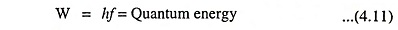where

• h is Planck’s constant and is equal to 6.626 x 10-34 joule-second and
• f is the frequency of light in hertz.

The energy required to liberate an electron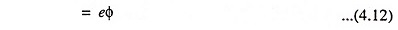where

• e = electronic charge and
• Φ is the work function in electron volts.

Hence for a photon to cause emission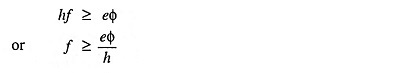The minimum frequency which can cause photoemission is called the threshold frequency and is given by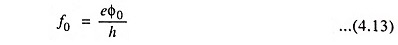Now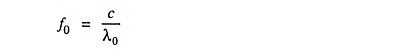where

• c is the velocity of light and is equal to 3 x 108 m/s.

Hence ‘threshold wavelength’ or ‘long wavelength limit’ is given by the equation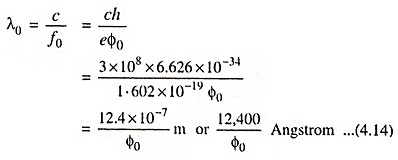If the radiation is of wavelength greater than that given by the above Eq. (4.14), then photoemission will not occur at all.

If the frequency of incident radiation is greater than f0 then incident radiation has more energy. This additional energy goes to impart kinetic energy to the emitted electrons. In that case, the energy relation becomes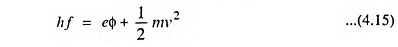where

• v is the maximum velocity of emission of the emitted electrons.

If V is the inhibiting voltage required to reduce the velocity of emitted electrons to zero then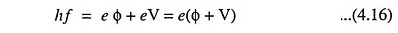The use of photoelectric emission phenomenon is made in phototubes which form the basis of TV and sound films.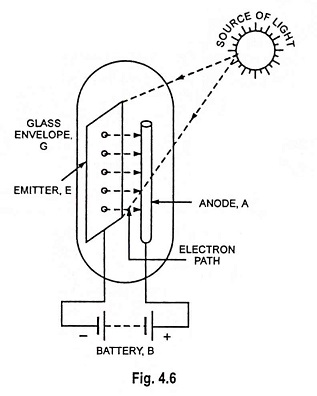The phenomenon of photoelectric emission is illustrated in Fig. 4.6. The emitter E and anode A are enclosed in an evacuated glass envelope G. The anode is a thin metal rod and is held at positive potential w.r.t. emitter. When light of suitable intensity and frequency falls on the surface of the emitter, electrons are ejected from the emitter surface. These electrons are attracted by the positive anode to constitute current in the external circuit. The flow of current will exist so long as illumination is maintained.

### Laws of Photoelectric Emission

1. Photoelectric emission from a metal surface takes place only when the energy content hf of the incident light exceeds the work function Φ of the metal.
2. The number of electrons emitted per second is directly proportional to the amount of incident light flux.
3. Maximum energy of emitted electrons is independent of the amount of incident light flux but is linearly related with the frequency of light flux.
Scroll to Top